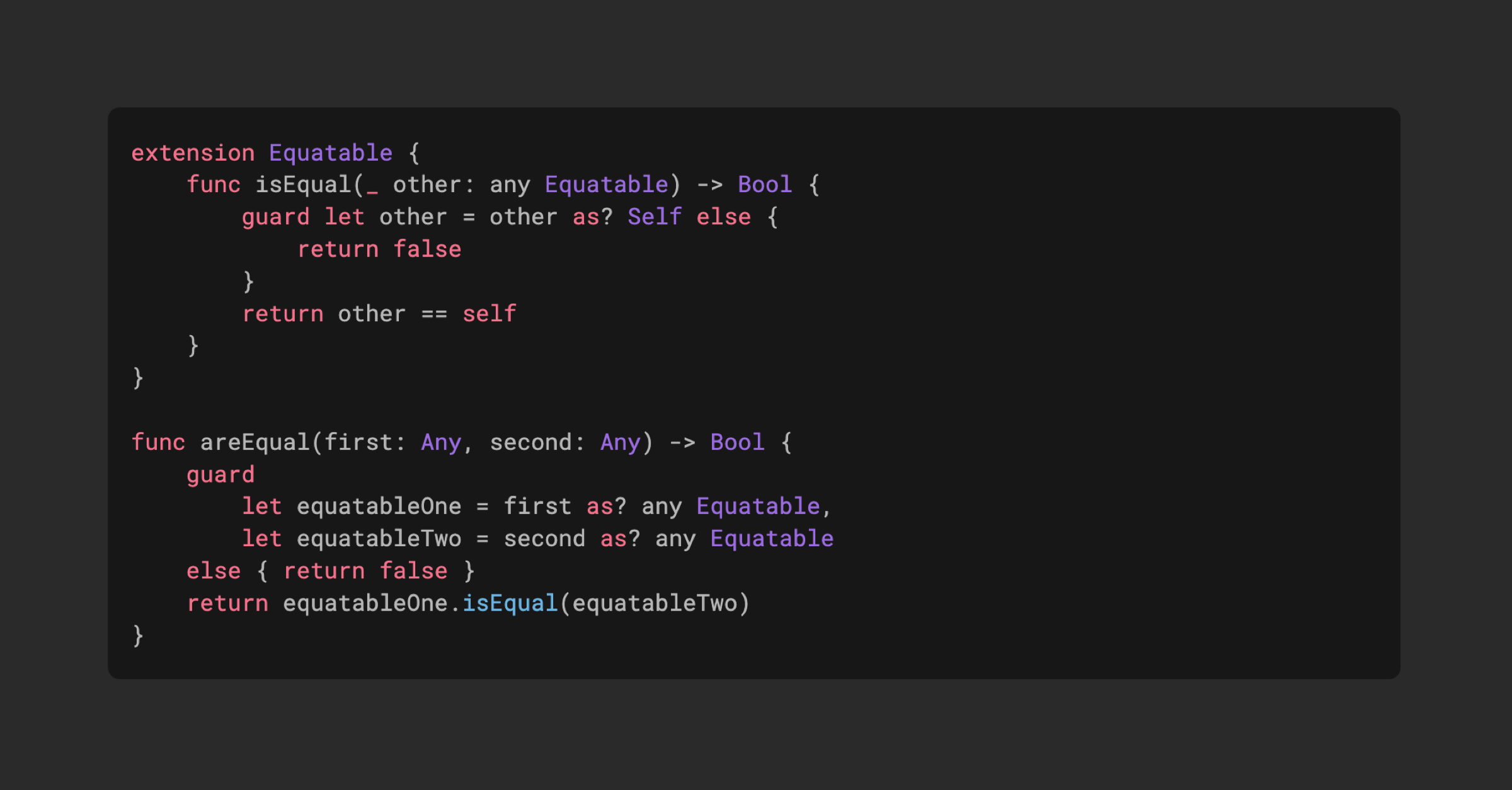# Check if two values of type Any are equal

0
18In Swift 5.7 that comes with Xcode 14 we can more easily check if two values ​​of type `Any` are equal, because we can cast values ​​to `any Equatable` and also use `any Equatable` as a parameter type thanks to Unlock existentials for all protocols change.

We can start by extending `Equatable` protocol with `isEqual()` method that accepts another `any Equatable` as an argument.

``````extension Equatable
func isEqual(_ other: any Equatable) -> Bool
guard let other = other as? Self else
return false

return other == self

``````

Inside `isEqual()` method we have access to `Self`so we can try to cast the `other` value to the same type as the concrete type of the value this method is called on. If the cast fails, the two values ​​cannot be equal, so we return `false`. If it succeeds, then we can check the two values ​​for equality, using `==` function on values ​​of the same concrete type.

Now we can define a global `areEqual()` function that will compare two values ​​of type `Any`. Unfortunately, we can not ‘extend `Any` type, so it will have to be a global function.

``````func areEqual(first: Any, second: Any) -> Bool
guard
let equatableOne = first as? any Equatable,
let equatableTwo = second as? any Equatable
else  return false

return equatableOne.isEqual(equatableTwo)

``````

Inside `areEqual()` we can try to cast both of `Any` values ​​to `any Equatable` and if we succeed, we can call our previously defined `isEqual()` method on one of the values, passing the other values ​​as an argument.

Overloading `==` operator for two values ​​of type `Any` seems to be causing a run-time crash because it enters a recursive loop in Swift at the moment, even if I put `@_disfavoredOverload` on it. When we have different overloads with parameters of concrete types and protocols, Swift can not always disambiguate them. `@_disfavoredOverload` should usually help with that, but it did not work in this case.

Another way to overload `==` operator would be to set generic parameters without constraints, so that Swift does not choose this version when it has two concrete types. This will let us compare two `Any` values ​​as well.

``````func ==<L, R>(lhs: L, rhs: R) -> Bool
guard
let equatableLhs = lhs as? any Equatable,
let equatableRhs = rhs as? any Equatable
else  return false

return equatableLhs.isEqual(equatableRhs)

``````

Now we can use our `areEqual()` function or `==` overload, when we need to compare two values ​​of type `Any`. For example, we might want to check if two dictionaries of `NSAttributedString` attributes are equal without casting them to `NSDictionary`.

``````typealias NSAttributes = [NSAttributedString.Key: Any]

func ==(lhs: NSAttributes, rhs: NSAttributes) -> Bool
if lhs.isEmpty && rhs.isEmpty  return true

guard lhs.count == rhs.count else  return false

for (key, lhsValue) in lhs
guard let rhsValue = rhs[key] else
return false

if !areEqual(first: lhsValue, second: rhsValue)
return false

return true

``````

Note, that the solution described here might hit some edge cases when working with class subtypes, as it was pointed out in this Twitter thread. And as Becca noted in this Twitter comment, `AnyHashable` has some tricks, like treating unbridged and bridged Foundation types as equal, that this will not handle.